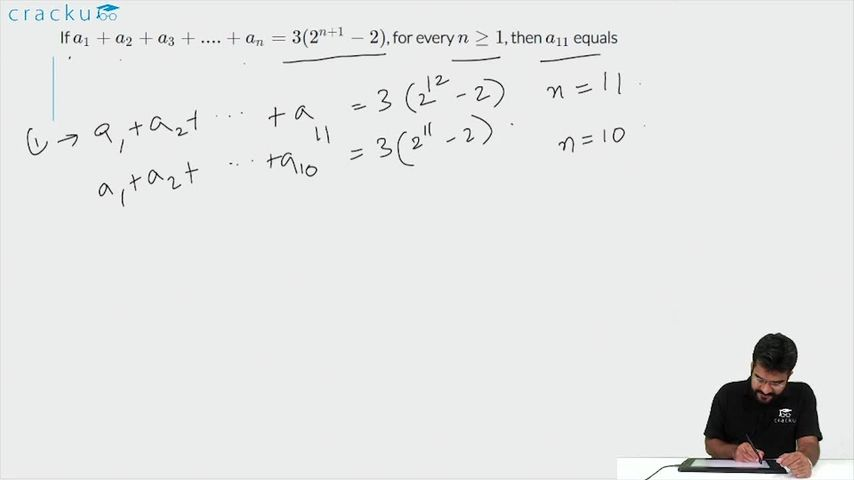Question 84

# If $$a_1 + a_2 + a_3 + .... + a_n = 3(2^{n + 1} - 2)$$, for every $$n \geq 1$$, then $$a_{11}$$ equals

Solution

11th term of series = $$a_{11}$$ = Sum of 11 terms - Sum of 10 terms = $$3(2^{11 + 1} - 2)$$-3$$(2^{10 + 1} - 2)$$

= 3$$(2^{12} - 2-2^{11} +2)$$=3$$(2^{11})(2-1)$$= 3*$$2^{11}$$ = 6144

### View Video Solution• All Quant CAT Formulas and shortcuts PDF
• 30+ CAT previous papers with solutions PDF